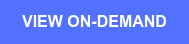<img height="1" width="1" style="display:none" alt="" src="https://www.facebook.com/tr?id=367542720414923&amp;ev=PageView&amp;noscript=1">### Join us wherever you are in the world. At home, in the office, on desktop, mobile or tablet for just 1.5 hours a day.

Insights you won't find anywhere else, brought directly to you.true true true true true true true true true true true true true true true true true true true true true true true true true true true true true true true true true true true true true true true true true true true true true true true true true true true true true true true true true true true true true true true true true true true true true true true true true true true true true true true true true true true true true true true true true true true true true true true true true